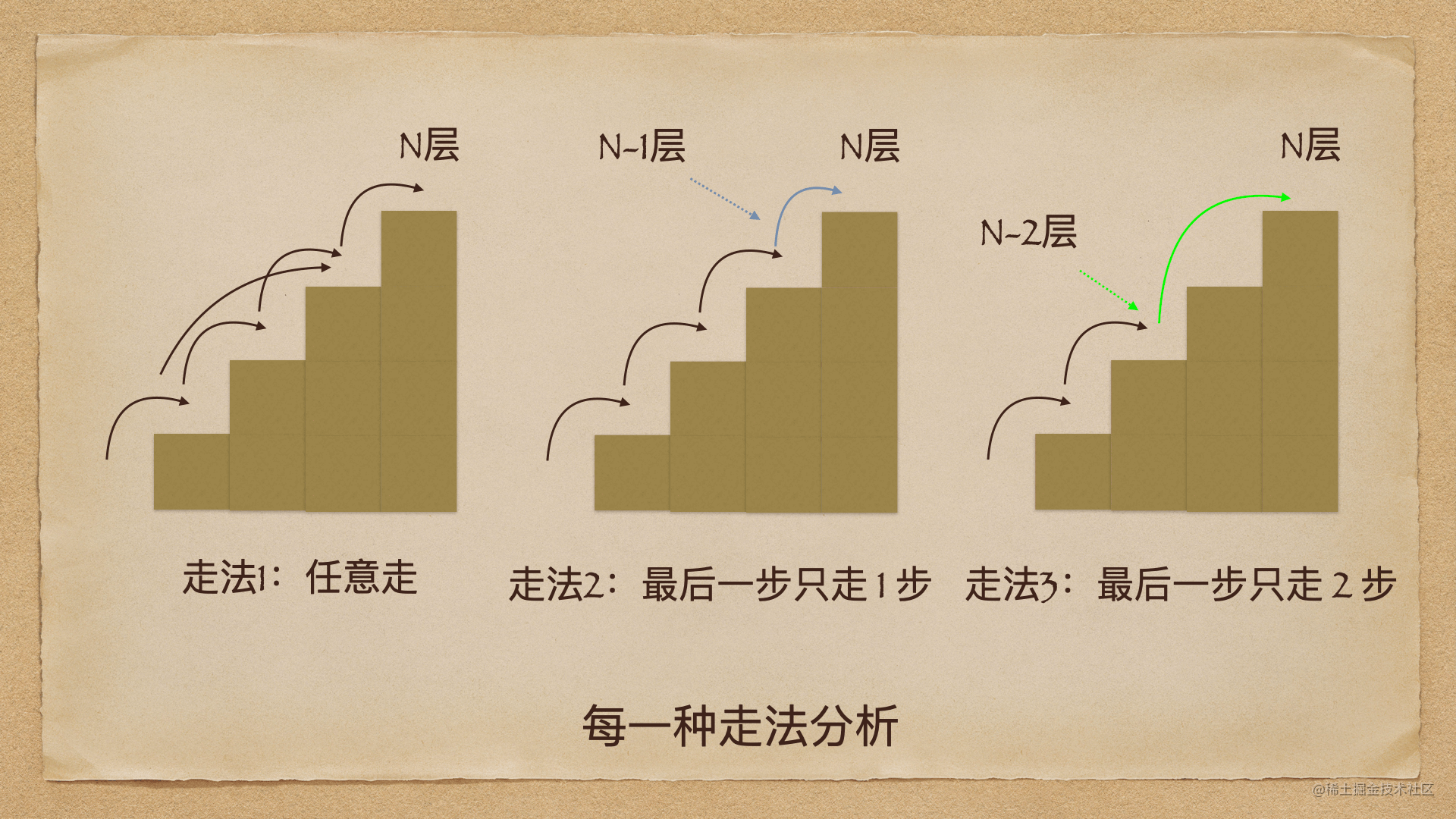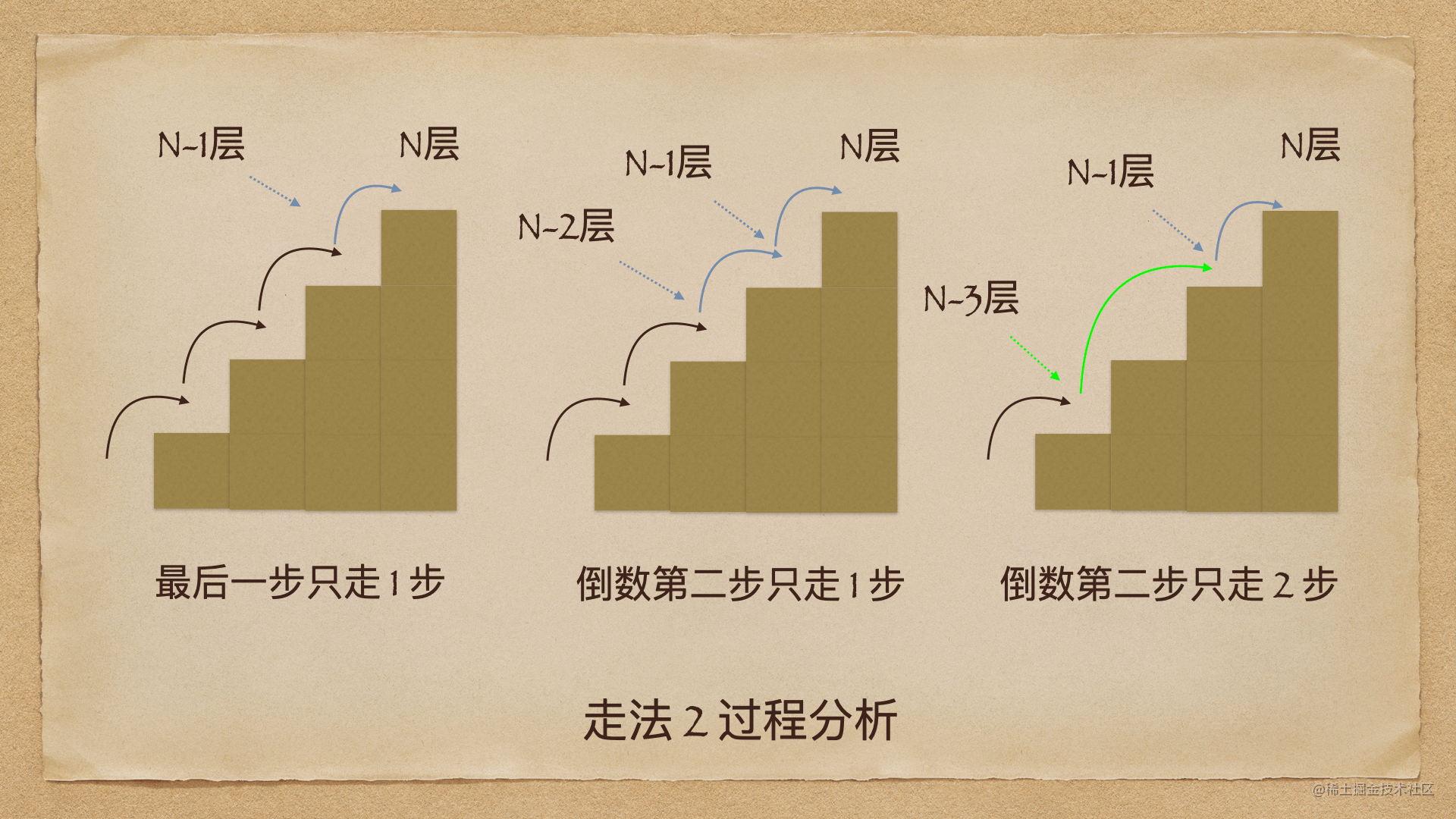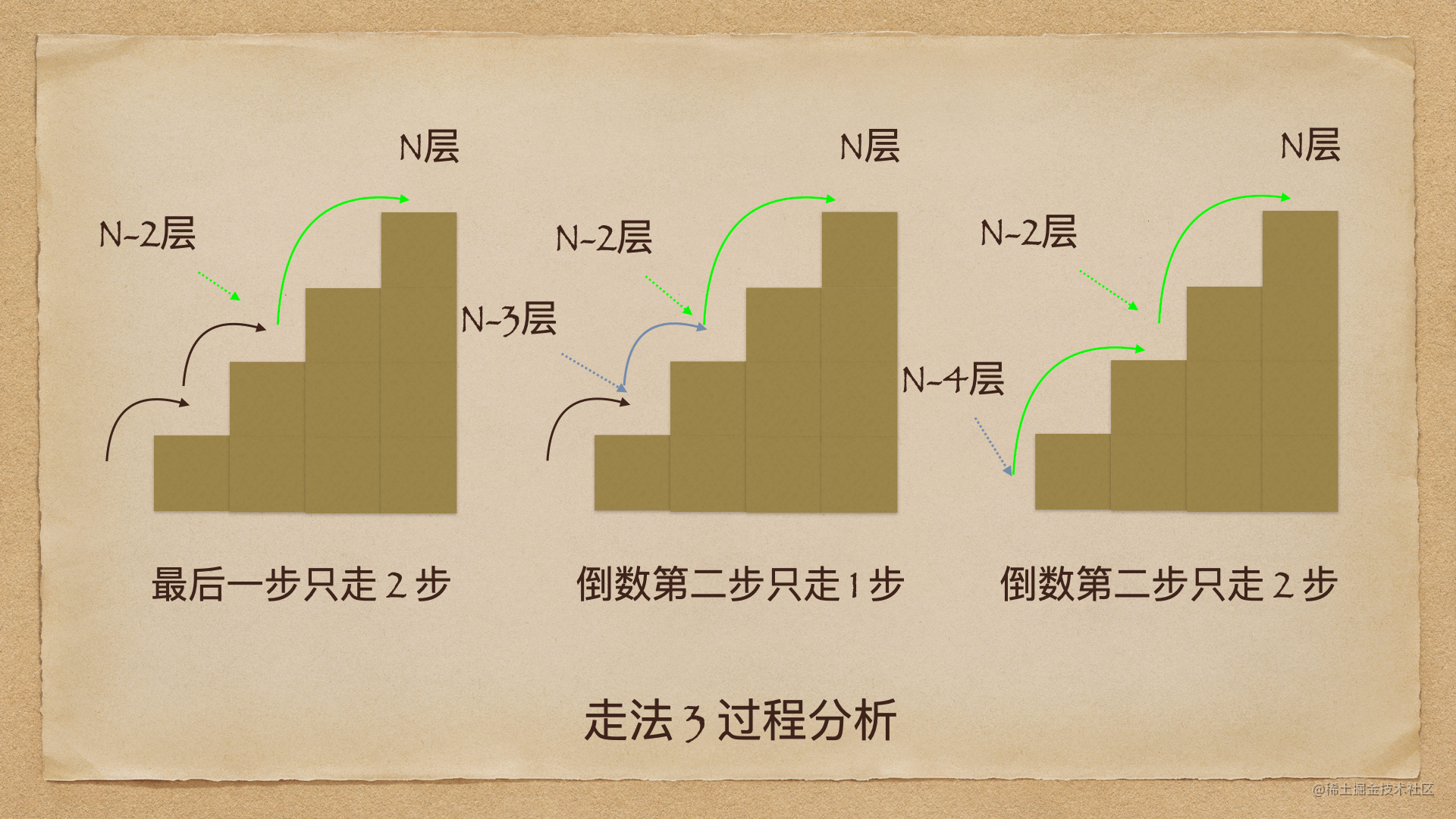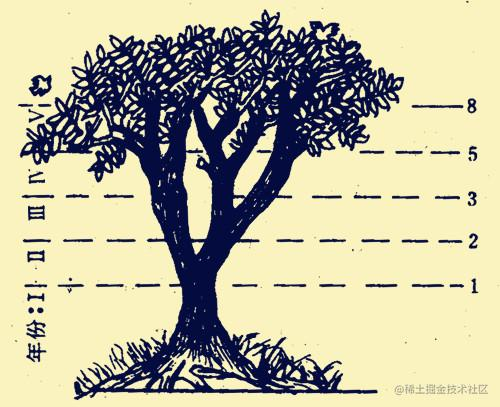# 图解算法 | LeetCode第 70 题爬楼梯问题## 流程分析

• 全程任意走，如全部 1 级走；
• 前面任意走，最后一步只走 1 级；
• 前面任意走，最后一步只走 2 级；• 当最后一步为 1 步时，即从 n-1 层开始；
• 当最后一步为 2 步时，即从 n-2 层开始；

## 归纳法分析

111
2211、2
33111、12、21
451111、112、121、211、22
5811111、1112、1121、1211、2111、221、212、122
.........

## 解法1：循环累加计算

``````const climbStairs = (n = 1) => {
if(n <= 2) return n;
let res = 0, n1 = 1, n2 = 2; // n1 表示前 2 项，n2 表示前 1 项
for(let i = 3; i<= n; i++){  // 前两项值固定，因此从第 3 项开始循环
res = n1 + n2;
n1 = n2;
n2 = res;
}
return res;
}

``````climbStairs(6); // 13

## 解法2：递归计算

``````const climbStairs = (n = 1) => {
if(n <= 2) return n;
return climbStairs(n-1) + climbStairs(n-2);
}

``````climbStairs(6); // 13

## 解法3：利用数组特性

``````const climbStairs = n => {
let result = [1,2];
for (let i = 2; i < n; i++) {
result.push(result[i-1] + result[i-2]);
}
return result[n-1];
};

## 解法4：利用 JavaScript ES6 新特性

``````const climbStairs = n => {
let a = b = 1;
for (let i = 0; i < n; i++) {
[a, b] = [b, a + b];
}
return a;
};

## 拓展知识：每次可以走 1 步、2 步、3 步

• 当最后一步为 1 步时，即从 n-1 层开始；
• 当最后一步为 2 步时，即从 n-2 层开始；
• 当最后一步为 3 步时，即从 n-3 层开始；

``````const climbStairs = (n = 1) => {
if(n <= 2) return n;
if(n == 3) return 4;
return climbStairs(n-1) + climbStairs(n-2) + climbStairs(n-3);
}

``````climbStairs(6); // 24

## 拓展知识：斐波那契数列（图片来源网络）（自然界中各种各样的裴波那契螺旋，图片来源于网络）